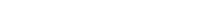# Math riddles Level 41 Answer with Hints and solutionsMath riddles game level 41 Answer with solution android game developed by Black games. Scroll Below to find details.

Math Riddles tests your IQ with mathematical puzzles. Challenge yourself with different levels of math puzzles and stretch the limits of your intelligence. Every IQ game is prepared with an approach of an IQ test. Improve your mathematics, challenge your brain with this game. There are many hard levels which you may not have solved then you can take help from here.

Math app level 41:

9,16=7

4,36=8

121,81=20

25,49=?Solution: the sum of the square roots of the two given numbers

5+7=12

If there is any Doubt/discrepancy then please let me know in comments or you are unable to understand solution then I will surely help you.

### One thought on “Math Riddles App Level 41 Solution Android”

•February 11, 2019 at 9:07 am